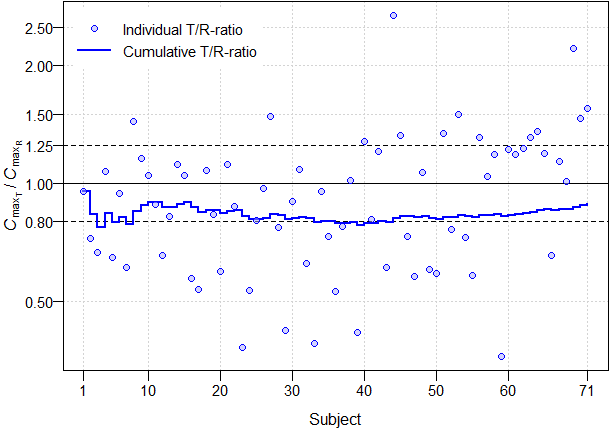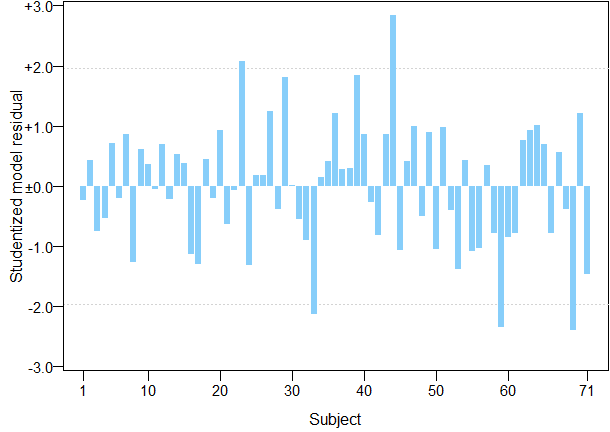## Bust the Buster [GxP / QC / QA]

Dear Ohlbe,

I tried to reproduce the ‘Buster routines’ of the paper mentioned above. As usual thescript at the end. Sorry, 200+ lines of code.

This is what I got for my manipulated data set:

ALike the paper’s Fig. 1.
We see that for the Test the deviations from the mean increase at the end.
However, statistically the trend is not significant.

BLike the paper’s Fig. 2.
For the reference all is good. As expected cause we use the original data.

COK – but the ratios start to rise (by chance) before the manipulation starts with subject 60.
Not convinced whether we can eye-ball it.

DLike the paper’s Fig. 3.
Possibly better. The original study was properly powered and by chance would pass with fewer subjects.
After the manipulation it needs almost the complete data set to pass.

ELike the paper’s Fig. 5.
Like in A we see rising residuals at the end. However, statistically the trend is not significant.

FLike the paper’s Fig. 4.
No clue.

GOnly for completeness.

For AC we need only the values of T and R and the subjects (more precisely: in the order of analyses). For the others additionally the randomization.
Potentially A, C, D (and E?) are useful. I have some doubts whether a ‘mild’ manipulation () like in my example can reliably be detected. You can try yet another data set where I multiplied T in the first half by 0.8 and in the second half by 1.25. Then the manipulation is obvious in C, D, and E (trend still not significant; p = 0.1677).

Unfortunately the data set BUa627311.csv is not accessible at doi:10.1016/j.ejps.2020.105595. I suspect that the manipulation was extreme.

library(PowerTOST) # Lazy: convert MSE to CV and back library(randtests) # Provides Wald-Wolfowitz runs test path <- "your.path" file <- "yourdata.csv" url  <- NULL # alternatively a valid url enclosed in single of double quotes # url <- "https://bebac.at/downloads/Post23021a.csv" # My original data # url <- "https://bebac.at/downloads/Post23021b.csv" # Manipulated data # Required case-sensitive column names (in any order): #   Subject, Sequence, Treatment, Period, Cmax sep  <- "," # Column separator: Inspect the file; alternatively "\t" or ";" dec  <- "." # Decimal separator; alternatively "," if (sep == dec) stop("sep and dec must must different!") com  <- "#" # For eventual comments, sometimes found in the header na   <- c("NA", "ND", ".", "", "Missing") # Will be set to NA if (sep == dec) stop("sep and dec must must different!") if (is.null(url)) { # Read local file   if (!substr(path, nchar(path), nchar(path)) == "/")     path <- paste0(path, "/") # Add trailing forward slash even on Windows   data <- read.csv(file = paste0(path, file), sep = sep, dec = dec,                    quote = "", strip.white = TRUE, comment.char = com,                    na.strings = na) } else {   data <- read.csv(file = url, sep = sep, dec = dec, quote = "",                    strip.white = TRUE, comment.char = com, na.strings = na) } subjects <- unique(data$Subject) n <- length(subjects) # If there are other columns in the file, drop them modeling <- FALSE modeling <- c("Subject", "Sequence", "Treatment", "Period", "Cmax") if (sum(names(data) %in% modeling) < 5) stop("Check column names!") data <- data[, modeling] modeling <- TRUE if (!modeling) { keep <- c("Subject", "Treatment", "Cmax") if (sum(names(data) %in% keep) < 3) stop("Check column names!") data <- data[, keep] } # Difference of Cmax from mean (both in log-scale) delta.T <- log(data$Cmax[data$Treatment == "T"]) - mean(log(data$Cmax[data$Treatment == "T"])) delta.R <- log(data$Cmax[data$Treatment == "R"]) - mean(log(data$Cmax[data$Treatment == "R"])) # Wald-Wolfowitz runs test of randomness for continuous data # alternative = "left.sided" tests for a trend rt.T <- runs.test(delta.T, alternative = "left.sided") rt.T <- c(paste(rt.T$runs, "runs"),              bquote(italic(p) == . (sprintf("%.4f", rt.T$p.value)))) rt.R <- runs.test(delta.R, alternative = "left.sided") rt.R <- c(paste(rt.R$runs, "runs"),              bquote(italic(p) == . (sprintf("%.4f", rt.R$p.value)))) # T/R-ratios: individual and cumulative with time Cmax.ratio <- data$Cmax[data$Treatment == "T"] / data$Cmax[data$Treatment == "R"] Cmax.cum <- numeric(n) for (i in 1:n) { Cmax.cum[i] <- prod(Cmax.ratio[1:i])^(1/i) } if (modeling) { # Only if we have Sequence and Period in the data res <- data.frame(subjects = NA_integer_, PE = rep(NA_real_, n-1), lower = NA_real_, upper = NA_real_, MSE = NA_real_, CV = NA_real_, power = NA_real_) # Factorize columns (effects) for the linear model facs <- c("Subject", "Sequence", "Treatment", "Period") data[facs] <- lapply(data[facs], factor) # Find the first occasion where we have at least one subject # in both sequences (otherwise, the model would no work) seqs <- character() for (subj in seq_along(subjects)) { seqs <- c(seqs, unique( as.character(data$Sequence[data$Subject == subjects[subj]]))) if (length(unique(seqs)) > 1) break } ow <- options("digits") options(digits = 12) # more digits for anova on.exit(ow) # ensure that options are reset if an error occurs for (i in subj:n) { tmp <- head(data, i * 2) res$subjects[i-1] <- length(unique(tmp$Subject)) # Acc. to GLs: Nested model is bogus; Subject works as well m <- lm(log(Cmax) ~ Sequence + Subject %in% Sequence + Period + Treatment, data = tmp) res$PE[i-1]       <- exp(coef(m)[["TreatmentT"]])     res[i-1, 3:4]     <- as.numeric(exp(confint(m, "TreatmentT",                                                 level = 1 - 2 * 0.05)))     res$MSE[i-1] <- anova(m)[["Residuals", "Mean Sq"]] res$CV[i-1]       <- mse2CV(res$MSE[i-1]) res$power[i-1]    <- suppressMessages(                            power.TOST(CV = res$CV[i-1], theta0 = res$PE[i-1],                                       n = length(unique(tmp$Subject)))) } res <- res[complete.cases(res), ] # Raw residuals of T pred <- suppressWarnings( exp(predict(m, newdata = data[data$Treatment == "T", 1:4])))   # Acc. to the paper. Commonly: residual = predicted – observed   resid    <- data[data$Treatment == "T", "Cmax"] - pred rt.resid <- runs.test(resid, alternative = "left.sided") rt.resid <- c(paste(rt.resid$runs, "runs"),                 bquote(italic(p) == . (sprintf("%.4f", rt.resid$p.value)))) # Studentized residuals stud <- rstudent(m) # Two values with opposite signs; we need only one of them stud <- stud[c(TRUE, FALSE)] # Uncomment the next line to show PE, CI, MSE, CV, post hoc power # print(round(res, 5)) } # Prepare plots x.tick <- pretty(1:n) x.tick <- c(1, x.tick[2:(length(x.tick)-2)], n) dev.new(width = 4.5 * sqrt(2), height = 4.5, record = TRUE) op <- par(no.readonly = TRUE) par(mar = c(3.2, 3.3, 0.1, 0.1), cex.axis = 0.9, mgp = c(2, 0.5, 0), ljoin = "mitre", lend = "square", ask = TRUE) ############################################### # Difference of log(Cmax) from geometric mean # ############################################### # ylim common to both treatments ylim <- c(-1, +1) * max(abs(range(c(delta.T, delta.R)))) y.ax <- sprintf("%+.1f", pretty(ylim)) y.ax[y.ax == "+0.0"] <- "±0.0" ######## # Test # ######## bp <- barplot(delta.T, plot = FALSE) plot(bp, bp, ylim = ylim, type = "n", axes = FALSE, frame.plot = TRUE, xlab = "Subject", ylab = expression(log(italic(C)[max[T]])*" – mean [ "* log(italic(C)[max[T]])*" ]")) barplot(delta.T, ylim = ylim, las = 1, col = "lightskyblue", border = NA, axes = FALSE, axisnames = FALSE, ylab = "", add = TRUE) axis(1, at = bp[x.tick], labels = x.tick) axis(2, at = pretty(ylim), labels = y.ax, las = 1) legend("top", legend = rt.T, inset = 0.02, , box.lty = 0, cex = 0.9, x.intersp = 0) ############# # Reference # ############# bp <- barplot(delta.R, plot = FALSE) plot(bp, bp, ylim = ylim, type = "n", axes = FALSE, frame.plot = TRUE, xlab = "Subject", ylab = expression(log(italic(C)[max[R]])*" – mean [ "* log(italic(C)[max[R]])*" ]")) barplot(delta.R, ylim = ylim, las = 1, col = "lightskyblue", border = NA, axes = FALSE, axisnames = FALSE, ylab = "", add = TRUE) axis(1, at = bp[x.tick], labels = x.tick) axis(2, at = pretty(ylim), labels = y.ax, las = 1) legend("top", legend = rt.R, inset = 0.02, , box.lty = 0, cex = 0.9, x.intersp = 0) ######################### # Individual T/R-ratios # ######################### plot(1:n, Cmax.ratio, type = "n", log = "y", axes = FALSE, xlab = "Subject", ylab = expression(italic(C)[max[T]]*" / "*italic(C)[max[R]])) y.tick <- sort(c(0.8, 1.25, axTicks(side = 2, log = TRUE))) axis(1, at = x.tick) axis(2, at = y.tick, las = 1) grid(nx = NA, ny = NULL) abline(v = x.tick, lty = 3, col = "lightgrey") abline(h = c(0.8, 1, 1.25), lty = c(2, 1, 2)) legend("topleft", legend = c("Individual T/R-ratio", "Cumulative T/R-ratio"), pch = c(21, NA), lty = c(0, 1), lwd = c(1, 2), inset = 0.02, col = c(rep("blue", 2)), pt.bg = "#AAAAFF80", box.lty = 0, pt.cex = 1, bg = "white", seg.len = 2.5, cex = 0.9, y.intersp = 1.25) box() lines(1:n, Cmax.cum, col = "blue", lwd = 2, type = "s") points(1:n, Cmax.ratio, pch = 21, col = "blue", bg = "#AAAAFF80") if (modeling) { ################ # PE and 90 CI # ################ ylim <- range(c(res$lower, res$upper), na.rm = TRUE) plot(1:n, Cmax.ratio, type = "n", ylim = ylim, log = "y", axes = FALSE, xlab = "Subject", ylab = "Point Estimate and 90% Confidence Interval") axis(1, at = x.tick) axis(2, at = y.tick, las = 1) grid(nx = NA, ny = NULL) abline(v = x.tick, lty = 3, col = "lightgrey") abline(h = c(0.8, 1, 1.25), lty = c(2, 1, 2)) legend("bottomright", legend = c("Point Estimate", "Confidence Limits"), lty = c(1, 1), lwd = c(2, 1), bg = "white", box.lty = 0, inset = 0.02, col = rep("blue", 2), seg.len = 2.5, cex = 0.9, y.intersp = 1.25) box() lines(res$subject, res$PE, col = "blue", lwd = 2, type = "s") lines(res$subject, res$lower, col = "blue", type = "s") lines(res$subject, res$upper, col = "blue", type = "s") ####### # MSE # ####### plot(res$subject, res$MSE, type = "n", xlim = c(1, n), axes = FALSE, xlab = "Subject", ylab = expression(italic(MSE))) axis(1, at = x.tick) axis(2, las = 1) grid(nx = NA, ny = NULL) abline(v = x.tick, lty = 3, col = "lightgrey") box() lines(res$subject, res\$MSE, col = "blue", lwd = 2, type = "s")   #############################   # Raw model residuals for T #   #############################   ylim <- c(-1, +1) * max(abs(range(resid)))   y.ax <- sprintf("%+.1f", pretty(ylim))   y.ax[y.ax == "+0.0"] <- "±0.0"   bp   <- barplot(resid, plot = FALSE)   plot(bp, resid, ylim = ylim, type = "n", axes = FALSE, frame.plot = TRUE,        xlab = "Subject", ylab = "Raw model residual (T)")   barplot(resid, ylim = ylim, col = "lightskyblue", border = NA,           axes = FALSE, axisnames = FALSE, ylab = "", add = TRUE)   axis(1, at = bp[x.tick], labels = x.tick)   axis(2, at = pretty(ylim), labels = y.ax, las = 1)   legend("top", legend = rt.resid, inset = 0.02, , box.lty = 0, cex = 0.9,          x.intersp = 0)   ###############################   # Studentized model residuals #   ###############################   ylim <- c(-1, +1) * max(abs(range(stud)))   y.ax <- sprintf("%+.1f", pretty(ylim))   y.ax[y.ax == "+0.0"] <- "±0.0"   bp   <- barplot(stud, plot = FALSE)   plot(bp, stud, ylim = ylim, type = "n", axes = FALSE, frame.plot = TRUE,        xlab = "Subject", ylab = "Studentized model residual")   abline(h = c(-1, +1) * qnorm(0.975), lty = 3, col = "lightgrey")   barplot(stud, ylim = ylim, col = "lightskyblue", border = NA,           axes = FALSE, axisnames = FALSE, ylab = "", add = TRUE)   axis(1, at = bp[x.tick], labels = x.tick)   axis(2, at = pretty(ylim), labels = y.ax, las = 1) } par(op)

Dif-tor heh smusma 🖖🏼 Довге життя Україна!Helmut SchützThe quality of responses received is directly proportional to the quality of the question asked. 🚮
Science QuotesIng. Helmut Schütz